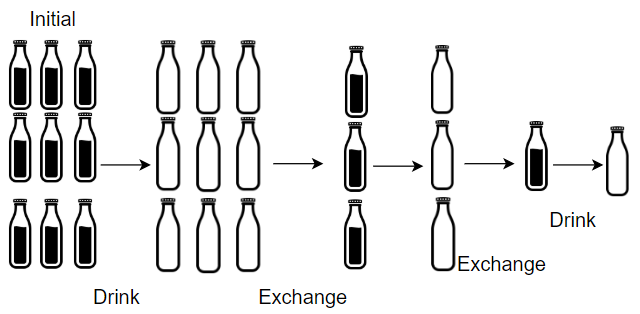##### Welcome to Subscribe On Youtube

Formatted question description: https://leetcode.ca/all/1518.html

# 1518. Water Bottles (Easy)

Given numBottles full water bottles, you can exchange numExchange empty water bottles for one full water bottle.

The operation of drinking a full water bottle turns it into an empty bottle.

Return the maximum number of water bottles you can drink.

Example 1:Input: numBottles = 9, numExchange = 3
Output: 13
Explanation: You can exchange 3 empty bottles to get 1 full water bottle.
Number of water bottles you can drink: 9 + 3 + 1 = 13.


Example 2:Input: numBottles = 15, numExchange = 4
Output: 19
Explanation: You can exchange 4 empty bottles to get 1 full water bottle.
Number of water bottles you can drink: 15 + 3 + 1 = 19.


Example 3:

Input: numBottles = 5, numExchange = 5
Output: 6


Example 4:

Input: numBottles = 2, numExchange = 3
Output: 2


Constraints:

• 1 <= numBottles <= 100
• 2 <= numExchange <= 100

Related Topics:
Greedy

## Solution 1.

• class Solution {
public int numWaterBottles(int numBottles, int numExchange) {
int total = 0;
int water = numBottles, empty = 0;
while (water > 0) {
total += water;
empty += water;
water = 0;
int exchange = empty / numExchange;
water += exchange;
empty -= numExchange * exchange;
}
}
}

############

class Solution {
public int numWaterBottles(int numBottles, int numExchange) {
int ans = numBottles;
while (numBottles >= numExchange) {
numBottles -= (numExchange - 1);
++ans;
}
return ans;
}
}

• // OJ: https://leetcode.com/problems/water-bottles/
// Time: O(log_exchange^bottle)
// Space: O(1)
class Solution {
public:
int numWaterBottles(int bottle, int exchange) {
int ans = 0, empty = 0;
while (bottle) {
ans += bottle;
empty += bottle;
int ex = empty / exchange;
empty -= ex * exchange;
bottle = ex;
}
return ans;
}
};

• class Solution:
def numWaterBottles(self, numBottles: int, numExchange: int) -> int:
ans = numBottles
while numBottles >= numExchange:
numBottles -= numExchange - 1
ans += 1
return ans


• func numWaterBottles(numBottles int, numExchange int) int {
ans := numBottles
for numBottles >= numExchange {
numBottles -= (numExchange - 1)
ans++
}
return ans
}

• /**
* @param {number} numBottles
* @param {number} numExchange
* @return {number}
*/
var numWaterBottles = function (numBottles, numExchange) {
let sum = numBottles;
while (numBottles >= numExchange) {
numBottles = numBottles - numExchange + 1;
sum++;
}
return sum;
};

• class Solution {
/**
* @param Integer $numBottles * @param Integer$numExchange
* @return Integer
*/
function numWaterBottles($numBottles,$numExchange) {
$sum =$numBottles;
while ($numBottles >=$numExchange) {
$numBottles =$numBottles - $numExchange + 1;$sum++;
}
return \$sum;
}
}